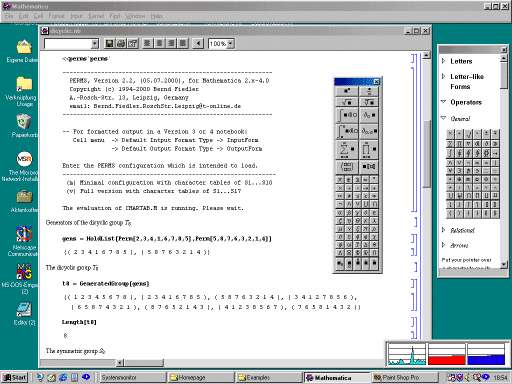# Examples of Calculations by means of PERMS

## Mathematica NotebooksHere we present a collection of Mathematica Notebooks which contain examples of calculations by means of the Mathematica package PERMS.

I compressed the Notebooks by gzip. They can be decompressed by the command

gzip -d -N name.gz  .

### Calculations by means of PERMS and Ricci

The following notebooks contain examples which were produced by a cooperation of PERMS and the Mathematica tensor package Ricci written by J. M. Lee.

### Combinatorial treatment of a normal form problem of vector analysis

The following notebooks deal with a normal form problem of the classical vector analysis. You can find details about this problem in the

that I gave at the 46. Seminaire Lotharingien de Combinatoire, Lyon, 2001. A table of the results is given in my list of mathematical tables on my home page. The calculations are stored in the following notebooks:

• Normal form problem of vector analysis - 4 vectors
• Normal form problem of vector analysis - 5 vectors
• Normal form problem of vector analysis - 6 vectors
• Normal form problem of vector analysis - 7 vectors
• Normal form problem of vector analysis - 8 vectors
• Normal form problem of vector analysis - 9 vectors

### Determination of the structure of algebraic curvature tensors by means of Young symmetrizers.

The following notebooks contain calculations which belong to the investigations of my paper .

### Generators of algebraic covariant derivative curvature tensors and Young symmetrizers Short formulas for algebraic covariant derivative curvature tensors

The following archive of 55 notebooks contains calculations which belong to the investigations of my papers  and . The archive is a WinZip archive which can be decompressed by means of WinZip or by the command "unzip dacurvnb.zip".